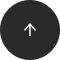Standard SQL

# How to Round Up a Number to the Nearest Integer in SQL

CEILING, CEIL

#### Problem:

You want to round up a number to the nearest integer.

#### Example:

Our database has a table named rent with data in the following columns: `id`, `city`, `area`, and `bikes_for_rent`.

idcityareabikes_for_rent
1Los Angeles1302.151000
2Phoenix1340.69500
3Fargo126.44101

Let’s show each city’s name along with the ratio of its area to the number of bikes for rent. This ratio should be an integer.

#### Solution:

```SELECT city, CEILING(area/bikes_for_rent) AS ratio
FROM rent;
```

The query returns each city with the ratio as an integer of rounded up the area per one bike.

idcityratio
1Los Angeles2
2Phoenix3
3Fargo2

#### Discussion:

Like its counterpart floor, ceiling is a mathematical operation that takes a number and rounds it up to the nearest integer. For example, the ceiling of 5 is 5, and so is the ceiling of 4.1.

SQL uses the `CEILING` function to perform this computation. It takes a single argument: the column whose values you’d like to round up to the nearest integer.

In our example, we’d like to calculate how many square meters (rounded up to the nearest integer) there are per one bike. In our example, we used `CEILING` like so: `CEILING(area/bikes_for_rent)`). This returns an integer result, not a float.

#### Recommended articles: